Working with Trigonometric Ratios

 When first learning about the Trigonometric Ratios, you will be working in Degree mode.  The calculator will default to Radian mode, so ....start by setting the calculator's MODE to Degree!!!!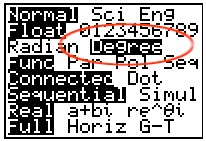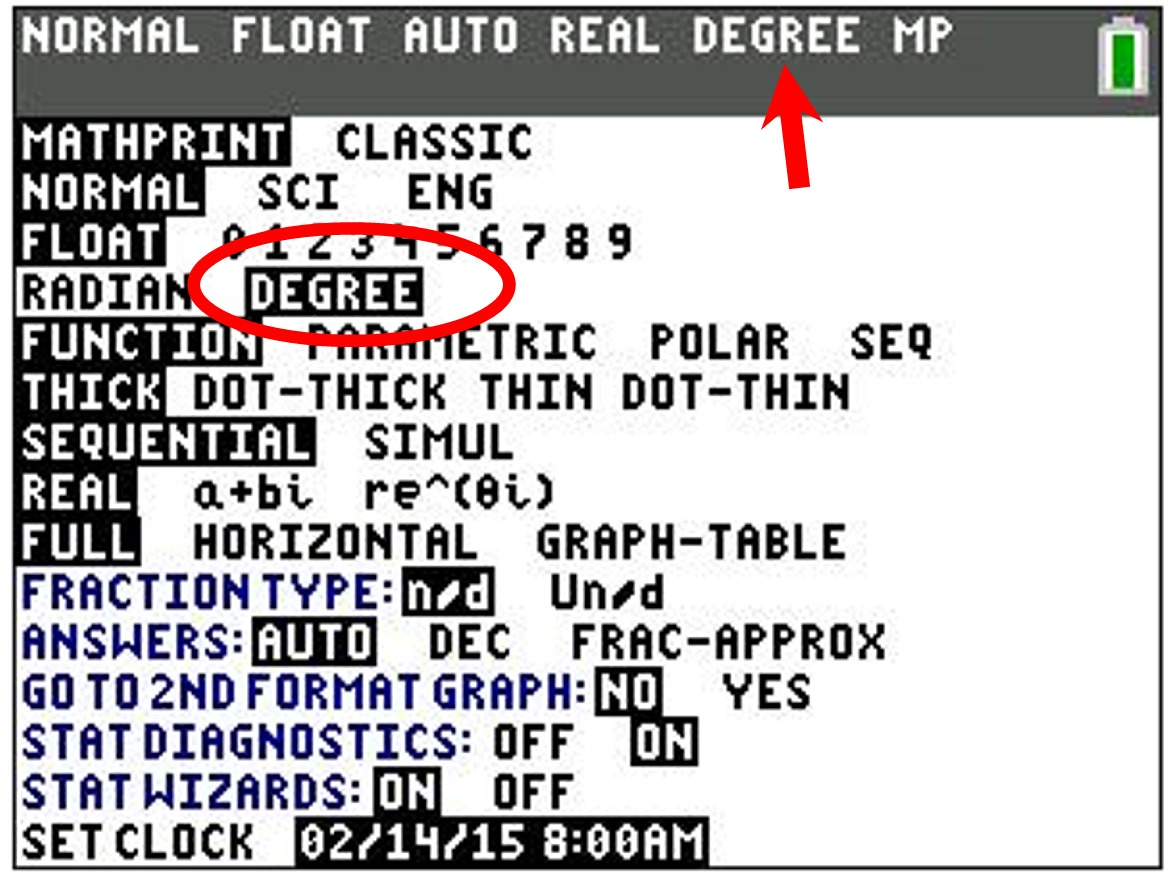Note:  If the calculator is set in Radian mode and you wish to find an angle in Degrees, you can insert a degree symbol after the number of degrees in the angle.  The degree symbol is found under 2nd APPS (Angle) #1 °. Only that one entry will be calculated in Degrees -- all others will remain in Radian mode.

 Find  sin 35°

Set
MODE

to
Degree
.

Type entry on the Home Screen.
Hit
ENTER.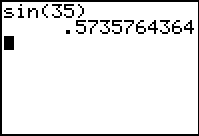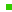You may be asked to round this answer to a specified value.If not asked to round, state the full screen value as the answer.If further calculations are needed, keep this full screen value and continue working.

 Find ∠A to the nearest degree, given cos A = 3/5

To find the number of degrees in the angle, you will need to use the calculator's inverse trigonometric functions. You will find these inverse functions (sin-1, cos-1, and tan-1) "above" the keys for sin, cos, and tan.

Set
MODE

to
Degree
.

On the Home Screen,
hit 2nd  cos  to get the
cos-1.
Type 3/5.    Hit
ENTER.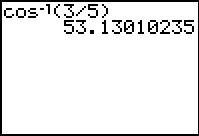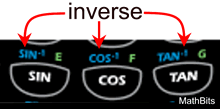Rounding answer to the nearest degree gives 53°.Finding Your Way Around TABLE of  CONTENTS